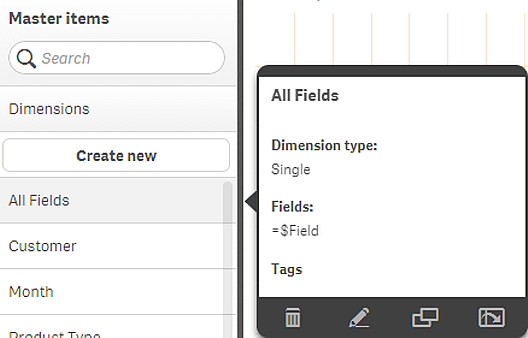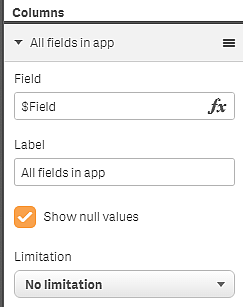# Tuning and improving visualizations

There are certain conventions and limitations you need to be aware of when working with Qlik Sense. For example: the maximum number of characters to use in names, descriptions, and expressions, as well as characters reserved for use by Qlik Sense only.

## Max number of visualizations

The maximum number of visualizations there can be on a sheet is limited to the number of cells on a sheet: 288 (24x12). The maximum practical number will be less than this because of the limited use for visualizations that are made up of only 1 cell.

## Upper limits on name lengths

The following limits apply to the number of characters that can be used in various situations in Qlik Sense:

Upper limits on name lengths
Situation Upper limit
Names (title, dimension, footnote...) Max 255 characters.
Descriptions Max 512 characters.
Expressions Max 64,000 characters.
Tags Max 31 characters per tag and max 30 tags per master item.

Text & image chart:

Max 12,000 characters.

## Naming conventions

You can refer to a number of entities by their names in Qlik Sense, such as:

• Fields
• Dimensions
• Measures
• Variables
• Bookmarks

Some characters are reserved for system purposes in Qlik Sense. To avoid potential errors, avoid using the following characters in names:

• :
• =
• [
• ]
• {
• }
• \$
• ´
• `
• '

If you use long names for your dimensions and measures they will be displayed truncated. “...” will be shown to denote that part of the name is hidden.

## Max number of characters in expressions

The maximum number of characters that can be written in a visualization expression is 64,000. If you attempt to build an expression with more than this number, the expression will be truncated.

## Conventions for number and time formats

In many interpretation and formatting functions it is possible to set the format for numbers and dates by using a format code. This topic describes the conventions used to format a number, date, time, or time stamp. These conventions apply both to script and chart functions.

## Number formats

To denote a specific number of digits, use the symbol "0" for each digit.

To denote a possible digit to the left of the decimal point, use the symbol "#".

To mark the position of the thousands separator or the decimal separator, use the applicable thousands separator and the decimal separator.

The format code is used for defining the positions of the separators. It is not possible to set the separator in the format code. Use the DecimalSep and ThousandSep variables for this in the script.

It is possible to use the thousand separator to group digits by any number of positions, for example, a format string of "0000-0000-0000" (thousand separator="-") could be used to display a twelve-digit part number as "0012-4567-8912".

Examples:

Example of number formats
Number format Description
# ##0

describes the number as an integer with a thousands separator. In this example " " is used as a thousands separator.

###0

describes the number as an integer without a thousands separator.

0000 describes the number as an integer with at least four digits. For example, the number 123 will be shown as 0123.
0.000

describes the number with three decimals. In this example "." is used as a decimal separator.

## Special number formats

Qlik Sense can interpret and format numbers in any radix between 2 and 36 including binary, octal and hexadecimal. It can also handle roman formats.

Special number formats
Format Description
Binary format

To indicate binary format the format code should start with (bin) or (BIN).

Octal format

To indicate octal format the format code should start with (oct) or (OCT).

To indicate hexadecimal format the format code should start with (hex) or (HEX). If the capitalized version is used A-F will be used for formatting (for example 14FA). The non-capitalized version will result in formatting with a-f (for example 14fa). Interpretation will work for both variants regardless of the capitalization of the format code.

Decimal format

The use of (dec) or (DEC) to indicate decimal format is permitted but unnecessary.

To indicate a format in any radix between 2 and 36 the format code should start with (rxx) or (Rxx) where xx is the two-digit number denoting the radix to be used. If the capitalized R is used letters in radices above 10 will be capitalized when Qlik Sense is formatting (for example 14FA). The non-capitalized r will result in formatting with non-capital letters (for example 14fa). Interpretation will work for both variants regardless of the capitalization of the format code. Note that (r02) is the equivalent of (bin), (R16) is the equivalent of (HEX), and so on.

Roman format

To indicate roman numbers the format code should start with (rom) or (ROM). If the capitalized version is used capital letters will be used for formatting (for example MMXVI). The non-capitalized version will result in formatting with lower cap letters (mmxvi). Interpretation will work for both variants regardless of the capitalization of the format code. Roman numbers are generalized with minus sign for negative numbers and 0 for zero. Decimals are ignored with roman formatting.

Examples:

Examples of special number formats
Example Result

num(199, '(bin)')

returns 11000111

num(199, '(oct)')

returns 307

num(199, '(hex)')

returns c7

num(199, '(HEX)' )

returns C7

num(199, '(r02)' )

returns 11000111

num(199, '(r16)')

returns c7

num(199, '(R16)' )

returns C7

num(199, '(R36)')

returns 5J

num(199, '(rom)')

returns cxcix

num(199, '(ROM)' )

returns CXCIX

## Dates

You can use the following symbols to format a date. Arbitrary separators can be used.

Symbols to format a date
Symbols Description
D

To describe the day, use the symbol "D" for each digit.

M

To describe the month number, use the symbol "M".

Use "M" or "MM" for one or two digits.

"MMM" denotes short month name in letters as defined by the operating system or by the override system variable MonthNames in the script.

"MMMM" denotes long month name in letters as defined by the operating system or by the override system variable LongMonthNames in the script.

Y

To describe the year, use the symbol "Y" for each digit.

W

To describe the weekday, use the symbol "W".

"W" will return the number of the day (for example 0 for Monday) as a single digit.

"WW" will return the number with two digits (e.g. 02 for Wednesday).

"WWW" will show the short version of the weekday name (for example Mon) as defined by the operating system or by the override system variable DayNames in the script.

"WWWW" will show the long version of the weekday name (for example Monday) as defined by the operating system or by the override system variable LongDayNames in the script.

Examples: (with 31st March 2013 as example date)

Examples of date formats
Example Result
YY-MM-DD describes the date as 13-03-31.
YYYY-MM-DD describes the date as 2013-03-31.
YYYY-MMM-DD describes the date as 2013-Mar-31.
DD MMMM YYYY describes the date as 31 March 2013.
M/D/YY describes the date as 3/31/13.
W YY-MM-DD describes the date as 6 13-03-31.
WWW YY-MM-DD describes the date as Sat 13-03-31.
WWWW YY-MM-DD describes the date as Saturday 13-03-31.

## Times

You can use the following symbols to format a time. Arbitrary separators can be used.

Symbols to format a time
Symbols Description
h

To describe the hours, use the symbol "h" for each digit.

m

To describe the minutes, use the symbol "m" for each digit.

s

To describe the seconds, use the symbol "s" for each digit.

f To describe the fractions of a second, use the symbol "f" for each digit.
tt

To describe the time in AM/PM format, use the symbol "tt" after the time.

Examples: (with 18.30 as example time):

Examples of time formats
Example Result
hh:mm describes the time as 18:30
hh.mm.ss.ff describes the time as 18.30.00.00
hh:mm:tt describes the time as 06:30:pm

## Time stamps

The same notation as that of dates and times above is used in time stamps.

Examples: (with 31th March 2013 18.30 as example time stamp):

Examples of time stamp formats
Example Result
YY-MM-DD hh:mm describes the time stamp as 13-03-31 18:30.
M/D/Y hh.mm.ss.ffff describes the time stamp as 3/31/13 18.30.00.0000.

## Using system fields in a visualization

You can use system fields in a visualization. System fields are created by Qlik Sense when the data load script is generated, and include information about the fields and tables in the loaded data. A system field begins with "\$", and you need to reference it by typing the field name including the "\$" manually. You can use a system field to create a dimension either as a master item or from the properties panel.

Preview of a dimension based on a system field .A system field added as dimension in the properties panel.s